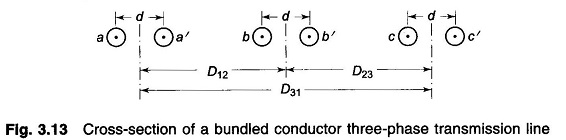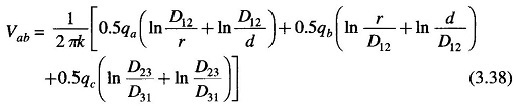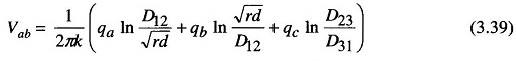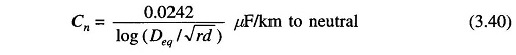## Use of Bundled Conductors in Transmission Line:

A Bundled Conductors in Transmission Line is shown in Fig. 3.13. The conductors of any one bundle are in parallel, and it is assumed that the charge per bundle divides equally among the conductors of the bundle as D12 ≫ d. Also     D12 – d ≈ D12 + d ≈ D12 for the same reason.The results obtained with these assumptions are fairly accurate for usual spacings. Thus if the charge on phase a is qa, the conductors a and a’ have a charge of qa/2 each; similarly the charge is equally divided for phases b and c.

Now, writing an equation for the voltage from conductor a to conductor b, we getorConsidering the line to be transposed and proceeding in the usual manner, the final result will beIt is obvious from Eq. (2.42) that the method of modified GMD is equally valid in this case.

Scroll to Top